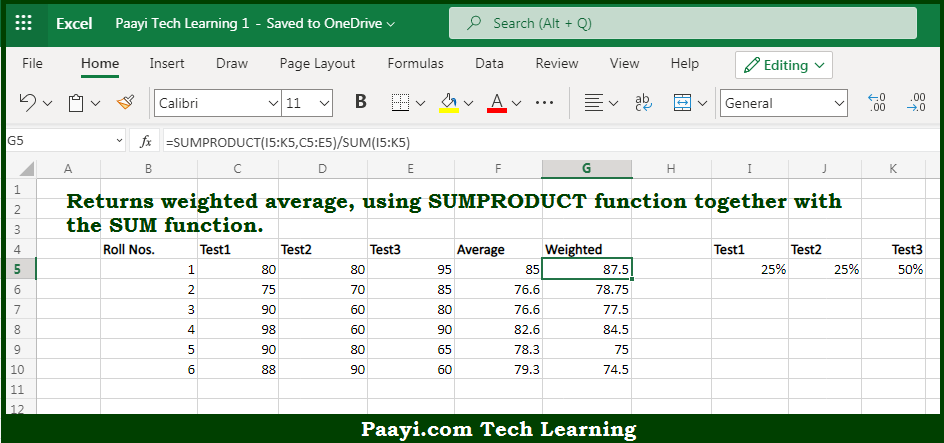# Learn How to Weighted Average in Microsoft Excel

Written by | 0 Comments | 512 Views

In this article, you will learn how to Weighted Average in Microsoft Excel using a single/combination(s) of functions. You will also know how to Weighted Average and see the generic formula.

Weighted Average in Microsoft Excel

The main purpose of this formula is to return the calculated weighted average. Here we will learn how to get the weighted average in the given range in the workbook in Microsoft Excel. That implies, with the help of a formula based on the SUMPRODUCT and SUM function you can able to return the calculated weighted average. So, with the help of this formula, you can able to get the weighted average in the given range in the workbook in Microsoft Excel.

General Formula to Weighted Average

=SUMPRODUCT(weights,values)/SUM(weights)

The Explanation for the Weighted AverageSo we know that with the help of the given formula above you can able to return the calculated weighted average. Here we will learn how to get the weighted average in the given range in the workbook in Microsoft Excel. As we know that the weighted average, also called a weighted mean, is an average where some values count more than others. It implies some values have more "weight". The weighted average by multiplying the values to the average by corresponding weights. After that dividing the sum of results by the sum of weights. So, with the help of this formula, you can able to return the calculated weighted average. Here we will learn how to get the weighted average in the given range in the workbook in Microsoft Excel.